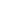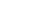How Cheenta works to ensure student success?
Explore the Back-Story

# Commutative does not commute in matrices: TIFR 2018 Part A, Problem 11# Understand the problem

[/et_pb_text][et_pb_text _builder_version="3.27" text_font="Raleway||||||||" background_color="#f4f4f4" box_shadow_style="preset2" custom_margin="10px||10px" custom_padding="10px|20px|10px|20px" hover_enabled="0" _i="1" _address="0.0.0.1"]True or False: Let A, B, C ∈ M3() be such that A commutes with B, B commutes with C and B is not a scalar matrix. Then A commutes with C. [/et_pb_text][/et_pb_column][/et_pb_row][et_pb_row _builder_version="3.25" _i="1" _address="0.1"][et_pb_column type="4_4" _builder_version="3.25" custom_padding="|||" custom_padding__hover="|||" _i="0" _address="0.1.0"][et_pb_accordion open_toggle_text_color="#0c71c3" _builder_version="3.27" toggle_font="||||||||" body_font="Raleway||||||||" text_orientation="center" custom_margin="10px||10px" hover_enabled="0" _i="0" _address="0.1.0.0"][et_pb_accordion_item title="Source of the problem" open="on" _builder_version="3.27" _i="0" _address="0.1.0.0.0" hover_enabled="0"]TATA INSTITUTE OF FUNDAMENTAL RESEARCH GS-2018 (Mathematics) [/et_pb_accordion_item][et_pb_accordion_item title="Topic" _builder_version="3.27" _i="1" _address="0.1.0.0.1" open="off" hover_enabled="0"]Linear Algebra [/et_pb_accordion_item][et_pb_accordion_item title="Difficulty Level" _builder_version="3.27" _i="2" _address="0.1.0.0.2" open="off" hover_enabled="0"]Medium [/et_pb_accordion_item][et_pb_accordion_item title="Suggested Book" _builder_version="3.27" _i="3" _address="0.1.0.0.3" open="off" hover_enabled="0"]Linear Algebra Hoffman and Kunze [/et_pb_accordion_item][/et_pb_accordion][et_pb_text _builder_version="3.23.3" text_font="Raleway|300|||||||" text_text_color="#ffffff" header_font="Raleway|300|||||||" header_text_color="#e2e2e2" background_color="#0c71c3" border_radii="on|5px|5px|5px|5px" box_shadow_style="preset3" custom_margin="48px||48px" custom_padding="20px|20px|20px|20px" _i="1" _address="0.1.0.1"]

[/et_pb_text][et_pb_tabs active_tab_background_color="#0c71c3" inactive_tab_background_color="#000000" _builder_version="3.27" tab_text_color="#ffffff" tab_font="||||||||" background_color="#ffffff" hover_enabled="0" _i="2" _address="0.1.0.2"][et_pb_tab title="Hint 0" _builder_version="3.22.4" _i="0" _address="0.1.0.2.0"]Do you really need a hint? Try it first!

[/et_pb_tab][et_pb_tab title="Hint 1" _builder_version="3.27" _i="1" _address="0.1.0.2.1" hover_enabled="0"]First we need to get some idea whether or not this may be true or false  . As a result we need to make some calculations. The first step to approach is always to build the expression of AC - CA & then see whether it is zero or not [/et_pb_tab][et_pb_tab title="Hint 2" _builder_version="3.27" _i="2" _address="0.1.0.2.2" hover_enabled="0"]Given AB = BA   &  BC = CB . Prove the following ! B(AC) = AC(B) & B(CA) = (CA)B and then subtract to get ( AC - CA )B =  B(AC -CA) Now this is not obvious if DB = BD & B being non- scalar matrix then D = 0 is too strong statement to be true  So this gives us idea  that it maybe false . Now to prove it false we need to construct a counter example [/et_pb_tab][et_pb_tab title="Hint 3" _builder_version="3.27" _i="3" _address="0.1.0.2.3" hover_enabled="0"]We can take approach using beautiful fact of matrices that they are transformation of spaces . Now given they are transformation of spaces and this is sort of abelian character showing up , we seek help from Groups     [/et_pb_tab][et_pb_tab title="Hint 4" _builder_version="3.27" _i="4" _address="0.1.0.2.4" hover_enabled="0"]You know why B is restricted to be non scalar because they form the centre of the(F) So we approach it in the following way If we can find a group of 3 x 3 matrices with non trivial centre . If we search for centre of groups then the only example available to us is Heisenberg Group In mathematics, the Heisenberg group, named after Werner Heisenberg, is the group of 3×3 upper triangular matrices of the formunder the operation of matrix multiplication. Elements a, b and c can be taken from any commutative ring with identity, often taken to be the ring of real numbers(resulting in the "continuous Heisenberg group") or the ring of integers (resulting in the "discrete Heisenberg group"). The continuous Heisenberg group arises in the description of one-dimensional quantum mechanical systems, especially in the context of the Stone–von Neumann theorem. More generally, one can consider Heisenberg groups associated to n-dimensional systems, and most generally, to any symplectic vector space. [/et_pb_tab][/et_pb_tabs][et_pb_text _builder_version="3.23.3" text_font="Raleway|300|||||||" text_text_color="#ffffff" header_font="Raleway|300|||||||" header_text_color="#e2e2e2" background_color="#0c71c3" border_radii="on|5px|5px|5px|5px" box_shadow_style="preset3" custom_margin="48px||48px" custom_padding="20px|20px|20px|20px" _i="3" _address="0.1.0.3"]

# Understand the problem

[/et_pb_text][et_pb_text _builder_version="3.27" text_font="Raleway||||||||" background_color="#f4f4f4" box_shadow_style="preset2" custom_margin="10px||10px" custom_padding="10px|20px|10px|20px" hover_enabled="0" _i="1" _address="0.0.0.1"]True or False: Let A, B, C ∈ M3() be such that A commutes with B, B commutes with C and B is not a scalar matrix. Then A commutes with C. [/et_pb_text][/et_pb_column][/et_pb_row][et_pb_row _builder_version="3.25" _i="1" _address="0.1"][et_pb_column type="4_4" _builder_version="3.25" custom_padding="|||" custom_padding__hover="|||" _i="0" _address="0.1.0"][et_pb_accordion open_toggle_text_color="#0c71c3" _builder_version="3.27" toggle_font="||||||||" body_font="Raleway||||||||" text_orientation="center" custom_margin="10px||10px" hover_enabled="0" _i="0" _address="0.1.0.0"][et_pb_accordion_item title="Source of the problem" open="on" _builder_version="3.27" _i="0" _address="0.1.0.0.0" hover_enabled="0"]TATA INSTITUTE OF FUNDAMENTAL RESEARCH GS-2018 (Mathematics) [/et_pb_accordion_item][et_pb_accordion_item title="Topic" _builder_version="3.27" _i="1" _address="0.1.0.0.1" open="off" hover_enabled="0"]Linear Algebra [/et_pb_accordion_item][et_pb_accordion_item title="Difficulty Level" _builder_version="3.27" _i="2" _address="0.1.0.0.2" open="off" hover_enabled="0"]Medium [/et_pb_accordion_item][et_pb_accordion_item title="Suggested Book" _builder_version="3.27" _i="3" _address="0.1.0.0.3" open="off" hover_enabled="0"]Linear Algebra Hoffman and Kunze [/et_pb_accordion_item][/et_pb_accordion][et_pb_text _builder_version="3.23.3" text_font="Raleway|300|||||||" text_text_color="#ffffff" header_font="Raleway|300|||||||" header_text_color="#e2e2e2" background_color="#0c71c3" border_radii="on|5px|5px|5px|5px" box_shadow_style="preset3" custom_margin="48px||48px" custom_padding="20px|20px|20px|20px" _i="1" _address="0.1.0.1"]

[/et_pb_text][et_pb_tabs active_tab_background_color="#0c71c3" inactive_tab_background_color="#000000" _builder_version="3.27" tab_text_color="#ffffff" tab_font="||||||||" background_color="#ffffff" hover_enabled="0" _i="2" _address="0.1.0.2"][et_pb_tab title="Hint 0" _builder_version="3.22.4" _i="0" _address="0.1.0.2.0"]Do you really need a hint? Try it first!

[/et_pb_tab][et_pb_tab title="Hint 1" _builder_version="3.27" _i="1" _address="0.1.0.2.1" hover_enabled="0"]First we need to get some idea whether or not this may be true or false  . As a result we need to make some calculations. The first step to approach is always to build the expression of AC - CA & then see whether it is zero or not [/et_pb_tab][et_pb_tab title="Hint 2" _builder_version="3.27" _i="2" _address="0.1.0.2.2" hover_enabled="0"]Given AB = BA   &  BC = CB . Prove the following ! B(AC) = AC(B) & B(CA) = (CA)B and then subtract to get ( AC - CA )B =  B(AC -CA) Now this is not obvious if DB = BD & B being non- scalar matrix then D = 0 is too strong statement to be true  So this gives us idea  that it maybe false . Now to prove it false we need to construct a counter example [/et_pb_tab][et_pb_tab title="Hint 3" _builder_version="3.27" _i="3" _address="0.1.0.2.3" hover_enabled="0"]We can take approach using beautiful fact of matrices that they are transformation of spaces . Now given they are transformation of spaces and this is sort of abelian character showing up , we seek help from Groups     [/et_pb_tab][et_pb_tab title="Hint 4" _builder_version="3.27" _i="4" _address="0.1.0.2.4" hover_enabled="0"]You know why B is restricted to be non scalar because they form the centre of the(F) So we approach it in the following way If we can find a group of 3 x 3 matrices with non trivial centre . If we search for centre of groups then the only example available to us is Heisenberg Group In mathematics, the Heisenberg group, named after Werner Heisenberg, is the group of 3×3 upper triangular matrices of the formunder the operation of matrix multiplication. Elements a, b and c can be taken from any commutative ring with identity, often taken to be the ring of real numbers(resulting in the "continuous Heisenberg group") or the ring of integers (resulting in the "discrete Heisenberg group"). The continuous Heisenberg group arises in the description of one-dimensional quantum mechanical systems, especially in the context of the Stone–von Neumann theorem. More generally, one can consider Heisenberg groups associated to n-dimensional systems, and most generally, to any symplectic vector space. [/et_pb_tab][/et_pb_tabs][et_pb_text _builder_version="3.23.3" text_font="Raleway|300|||||||" text_text_color="#ffffff" header_font="Raleway|300|||||||" header_text_color="#e2e2e2" background_color="#0c71c3" border_radii="on|5px|5px|5px|5px" box_shadow_style="preset3" custom_margin="48px||48px" custom_padding="20px|20px|20px|20px" _i="3" _address="0.1.0.3"]

# Similar Problems

This site uses Akismet to reduce spam. Learn how your comment data is processed.

### Knowledge Partner